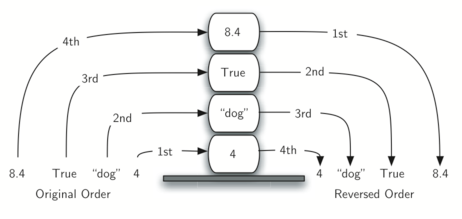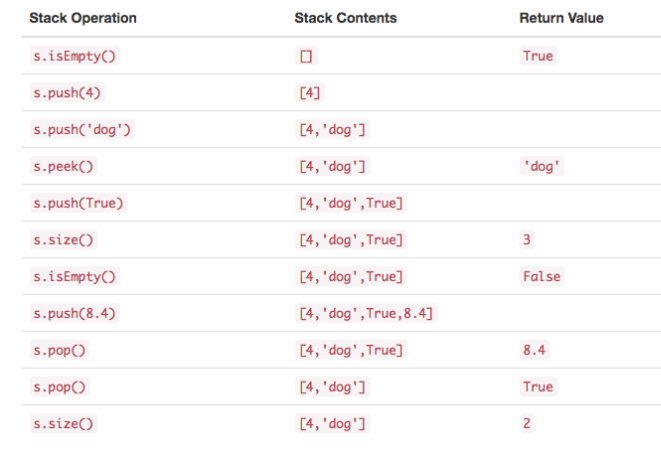Python 实现栈

1.什么是栈2.栈的基本操作

Stack() 创建一个空的新栈。 它不需要参数，并返回一个空栈。
push(item)将一个新项添加到栈的顶部。它需要 item 做参数并不返回任何内容。
pop() 从栈中删除顶部项。它不需要参数并返回 item 。栈被修改。
peek() 从栈返回顶部项，但不会删除它。不需要参数。 不修改栈。
isEmpty() 测试栈是否为空。不需要参数，并返回布尔值。
size() 返回栈中的 item 数量。不需要参数，并返回一个整数。3.python实现栈

class Stack:
def __init__(self):
self.items = []

def isEmpty(self):
return self.items == []

def push(self, item):
self.items.append(item)

def pop(self):
return self.items.pop()

def peek(self):
return self.items[len(self.items)-1]

def size(self):
return len(self.items)


from pythonds.basic.stack import Stack

s=Stack()

print(s.isEmpty())
s.push(4)
s.push('dog')
print(s.peek())
s.push(True)
print(s.size())
print(s.isEmpty())
s.push(8.4)
print(s.pop())
print(s.pop())
print(s.size())


http://www.pythonworks.org/pythonds